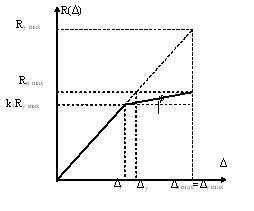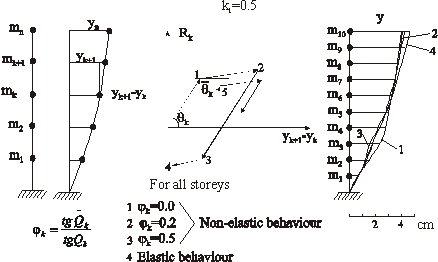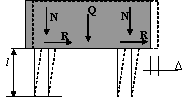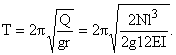Earthquake Engineering

УДК 624

Academician E. Y. Khachian, P. A. Ter-Petrosyan, V. V. Poghosyan

The limitation of the axial normal stresses in the reinforced concrete
structures under the fixed loads during the seismic impacts

(Submitted 8/VII 2003)

1. Introduction. Usually for the non-elastic deformation using the yielding coefficient m what is the relation of maximal limiting deformation in the elastic-plastic phase to deformation at the moment when plastic deformation starting (end of elastic deformation):
 m = Dn max Dy .
(1)
As shown on the figure 1 it takes place
 Dn max Dyў = Ry max Rn max .
(2)Figure 1. To definition of coefficient of damages.

For the ideal elastic-plastic materials (structures) Dy = Dyў and as result will have
 Rn max = Ry max m ,   or    Rn max  =  k1Ry max,
(3)
where is
 k1 = 1 m = Rn max Ry max .
(4)

Thus, the coefficient of damages k1 represents the relation of elastic-plastic response of system in the moment of beginning of plastic deformation Rn max to conventional maximal response Ry max of this system in the assumption of its elastic behavior from beginning to end of earthquake.
The non-elastic deformation in the buildings and structures always arise during the strong earthquake. The study of these deformations under the accelerograms of strong earthquakes for the different "regeneration force - relative movement" non-linear relations is shown one important property of the process of structure deformation: maximal displacement of the elastic and non-elastic structures with the same equivalent natural periods of free oscillation and damping characteristics very closed when the value of das not exceed 3-4 [1,2,3]. In the table 1 are shown the calculation results for simple system with one degree of freedom for elastic and equivalent non-elastic system under the different values of k1  under the accelerogram of real earthquake (the maximal ground acceleration is 0.25g, Eureka Earthquake, 21.12.1954), which are confirm above mentioned.

Table 1

The value of maximal displacements

The value of initial period of free oscillation, T, sec

Maximal displacement, cm

Elastic calculation

Non-elastic calculation

m=1.43
k1=0.7

m=2.0
k1=0.5

m=3.33
k1=0.3

0.35

2.41

2.37

1.99

2.89

0.4

2.97

3.91

3.67

2.94

0.45

3.97

3.87

4.14

2.86

0.5

4.27

4.18

3.41

3.83

0.55

3.01

3.45

3.81

3.99

0.6

5.53

5.34

5.04

6.6

0.7

6.05

4.98

6.33

4.54

0.8

4.57

4.02

3.40

4.83

1.0

3.86

3.44

3.91

4.10

1.2

2.96

3.61

4.99

3.28

1.5

8.04

8.55

7.67

7.12

2.0

14.3

14.4

14.0

13.9

2.5

17.2

18.0

19.8

11.3

3.0

18.5

18.6

15.7

12.7

The same results were received for the 10 storeys reinforced concrete frame building with masses m1 = m2 = ...m10 = 52kgsec2/cm, storeys stiffness a1 = a2 = ...an = 110400kg/cm and k1 = 0.5, jk = 0;0.2;0.5 under the calculation by accelerogram of Holister Earthquake (09.03.1949, maximal ground acceleration was 0.125g). The results of this calculation are shown in figure 2.
2. Criterion of seismic resistance for calculation by the Armenian Codes. The formation of damages in reality is the cause of decreasing the stiffness of structure, increasing the its period of natural oscillation and arising of residual deformation. The optimum design solution is the compromise of two approaches: decreasing of stiffness (through including the coefficient k1) and limitation of displacements (elastic + elastic-plastic).Figure.2 Distributing of displacements for elastic and non-elastic calculations
of 10 storeys building.

On the sample of system with one degree of freedom - one floor frame with rigid beam, carrying the concentrated load Q (figure 3) will show the conditions when it is possible to have above-mentioned compromise for design by the Armenian Norm CCRA II-2.02-94 .Figure 3. Deformation scheme of structure

According to code  the elastic seismic load for the one floor structure for the I-st category of soil and periods T і 0.4 sec will be
 S = QAk0b(T) = Q g t(T),
(5)
where is
 t(T) = 1.35Agk0 T2/3
(6)
is horizontal acceleration of concentrated mass Q/g.
Because of between concentrated mass and its displacement there is relation :
 t(T) = жзи 2p T цчш 2 y(T)
(7)
for displacement of system by norm CCRA II-2.02-94
 y(T) = tT2 (2p)2 = 1.35Agk0T2 (2p)2T2/3 .

If will take into consideration the seismic zone 3 (A=0.4) and taking k0=0.8 for I-st category of soil for maximal displacements under the elastic oscillation will have

 ymax(T) = 10.73T4/3.
(8)

The period of natural oscillations for the structural system (column with fixed edges), which is shown on the figure 2, will have(9)
Transfer (9) to (8) and assuming
 s = N bh ,    I = bh3 12
(10)
where is s normal stress in the columns under the permanent loading for the displacement (8) will have (in the cm)
 ymax = 1.27s2/3h-4/3l2E-2/3.
(11)

Thus we can take into consideration that during the earthquakes the expression (11) for the maximal displacements of structure for the conventional elastic and real elastic-plastic behavior is the same, where s is normal stress in the column, E - initial module of elasticity, h -column cross section height and l - column height.
For the optimal design it is necessary to determine by the experiment the value of very important parameter: what displacement limitation may be allow or the structure.
J. F. Borjes and A.Ravara have shown  on the base of experimental studying of reinforced concrete elements buckling and on the base of hypothesis of plane section and ideal diagram ßtress-deformation" for concrete and reinforcement (maximal relative deformation 1% for the strained reinforcement and 0.35% for the concrete correspond to destruction) and non-elastic behavior, that for the fixed by two edges column the boundary displacement express in the following way

 ylim = 2×10-4 l2 nh ,
(12)

where are n = [N/(bhsbk)], sbk - characteristically strength of cylindrical concrete samples with age 28 days, which is assume equal to 85% of cubic strength. The percentage of reinforcement of samples was in limitation 0.5-1.5%, and value of yielding coefficient equal m = 3.5. By the mean of authors , at the more large percentages of reinforcement the value of yielding coefficient may be too high. As authors mention the expression (12) may be true for different classes of concrete and steel.
Base on the main criterions of seismic resistance - the maximal movement of structure with taking into consideration of elastic-plastic deformation can't exceed permissible value:
 ymax < ylim
(13)
On the base of (11) and (12) will have:
 1.27s2/3h-4/3l2E-2/3 < 2×10-4 sbk sh l2.
(14)
From where
 slim < 5.23×10-3sbk3/5E2/5h1/5.
(14ў)

The expression (14) allows determine relation between the height of cross section h and minimal value of axial stress s under the permanent loading for the column with known concrete class (strength). On the case of keeping of this relation the value of yielding of structure m may be take equal 3.5 (k1=0.285).
3. Analysis of results. In the table 2 are shown boundary values of axial stresses slim lim what were calculated by (14) in dependence of column cross section h for the light and heavy concrete which are usually use in the construction of frame buildings in Armenia (concrete class B15, B20 and B25).

Table 2

The limit values of axial stresses

 Height of column cross section h, cm Limit values of axial stress slim under the permanent loading for the different concretes, kgf/cm2 light concrete heavy concrete B15 D2000 B20 D1800 B20 D2000 B25 D2000 B15 B20 B25 20 24.3 32.5 34.4 39.5 26.8 39.2 45.6 30 26.4 35.3 37.3 42.8 29.1 42.5 49.5 40 27.9 37.4 39.5 45.4 30.8 45.0 52.4 50 29.2 39.1 41.3 47.4 32.2 47.1 54.8 60 30.3 40.5 42.9 49.2 33.4 48.8 56.8 70 31.2 41.8 44.3 50.7 34.5 50.3 58.6 80 32.1 42.9 45.4 52.1 35.4 51.7 60.2

The values of characteristically strength of concrete sbk correspondingly take equal 200, 250, and 300kgf/cm2. For the light concrete were considered three class of concrete: B20 (D1800), B20 (D2000) and B25 (D2000) with initial module of elasticity E equal 173×103, 199×103 and 214×103 kgf/cm2 correspondingly. For the heavy concrete class B15, B20 and B25 the values of initial modules of elasticity were 235×103, 275×103 and 306×103 kgf/cm2 correspondingly .
As you can see from Table 2, with increasing of column cross section height h the value of boundary axial stress under the permanent loading also increase, but very slowly. For the column from light concrete at h=30ё50cm the value of slim is 13ё15% from sbk and at h=60ё80cm - 15ё16% from sbk. For the column from heavy concrete these relation is insignificant higher and there are at h=30ё50cm - 15ё19% from sbk and at h=60ё80cm - 18ё21% from sbk.
The limitation of relative drifr of stories D of buildings also can be considers as measure for the structure seismic resistance. According to Armenian Code CCRA II-2.02-94 for the frame reinforced concrete structures this limitation is 1/200 of the building height . With accordance to experimental results what were received by M. Melkumyan  for the P-type reinforced concrete frame the limit drift D may be assume a 1/200ё1/160 of the floor height. In accordance to  the relative displacement for the buildings with ordinary height of the floors cannot exceed 2cm, that is for floor height l = 300cm corresponded to D = l/150.
On the base of (11), the drift limitation can be express by the following way
 1.27s2/3h-4/3l2E-2/3 < D.
(15)
From (15) it is possible to determine the minimal height of column cross section for the different values of axial stresses s:
 h > 1.196s1/2E-1/2D-3/4l3/2.
(16)
For the above considered columns from the light and heavy concrete B15, B20 and B25 classes the minimal values of h calculated by (16) for the two values of limit drift D = 1.5 cm (l/200) and D = 2.0cm (l/150) are shown in the table 3.

Table 3

The minimal values of columns section height h

 Normal stress in the column under the permanent loading s, kgf/cm2 The height of column h must be not less than (cm) acceptable drift is D=1.5cm acceptable drift is D=2.0cm ight concrete heavy concrete light concrete heavy concrete B20 D1800 B20 D200 B25 D200 B15 B20 B25 B20 D1800 B20 D200 B25 D200 B15 B20 B25 5 25 23 22 21 20 18 20 19 18 17 16 15 10 35 33 31 30 28 26 28 26 25 24 22 21 15 43 40 38 37 34 32 34 32 31 29 27 26 20 49 46 44 42 39 37 40 37 35 34 31 30 25 55 51 49 47 44 41 44 41 39 38 35 33 30 60 56 54 52 48 45 47 45 43 42 38 36 35 65 61 59 56 52 49 52 49 46 45 42 40 40 69 65 63 60 55 52 56 52 51 48 44 42 45 74 69 66 63 59 55 59 56 53 51 47 44 50 79 73 70 67 62 58 63 58 56 54 50 47 55 82 76 73 70 65 61 66 61 59 56 52 49 60 85 80 77 73 68 64 69 64 62 59 54 52 70 92 86 83 79 73 69 74 69 67 64 56 56 80 98 92 87 85 78 74 79 74 70 68 60 60

As shown in table 3 the minimal height of the columns cross-section at D = 2cm in 1.24 times less than the acceptable distortion D = 1.5cm. The comparison of the data of tables 2 and 3 shown the significant differences between limit maximal axial stresses and minimal heights of columns sections for the limit drift calculated by formula (15) and deformations by formula (12). The values of axial stresses s for the small and large height of columns section change insignificantly when use the formula (12) and when use the formula (15) this change is more rapidly. But in real limitation of column cross section height 50 < h < 70cm the using of both formula give the same result.
4. Conclusion. The research shows the connections
between the coefficient of damaged, floors acceptable drifts, axial stresses and cross section height of reinforced concrete columns. In the research it is shown:
1) for the ordinary dimensions of cross section of columns (from 40 cm to 80 cm) and at the coefficient of damages k Ј 0.3 the values of axial stresses under the permanent loading can not be more than 25% from characteristically strength of concrete;
2) the minimal height of column cross section at the acceptable drift D = 2.0cm in the 1.24 times less than at D = 1.5cm;
3) the minimal height of cross section of column from heavy concrete is in the 1.5 times less than light concrete when the class of concrete is the same.
The main conclusion of research is that for the ordinary dimensions of columns cross sections the axial forces must be limited and its values must be not more than 25% from characteristically strength of concrete.
Above were shown the analysis of simple system with one degree of freedom. As shown in [2,3,9] and many other works, the maximal displacements in the elastic and elastic-plastic calculations of multi-storey buildings also insignificantly different like it is for above mentioned single floor buildings. In this case it is only necessary to increase the maximal displacement (8) on 20-25%. Therefore above-mentioned conclusions can be the same for ground floors of multi-storey buildings.
The selective calculations by norms of the former USSR (k1=0.24, A=0.1-0.2g) of axial stresses under the permanent loadings for existing in Armenia multi-storey frame buildings shown that they don't satisfy to above-mentioned conditions. It is significantly decrease their real yielding and increase the possibility of brittle destruction during the earthquakes with ground acceleration 0.3-0.4g, that foreseen by Armenian Codes.

Yerevan State University of Architecture and Construction

References

1. Newmark N.M., Rosenblueth E. Fundamentals of Earthquake Engineering, (Prentice-Hall, Inc.Englewood CCliffs, N.Y., 1971) translation on Russian, M., p.344, 1980.
2. Borges I. F., Ravara A. Earthquake Engineering Seismic Design of Reinforced Concrete Buildings, (Lisbon, 1969) translation on Russian, M., p.136, 1978.
3. Khachian E. E. Seismic Impact on High Buildings and Structures, Hayastan, Yerevan, p.328, 1973. (in Russian)
4. Seismic Construction. Construction Codes of Republic of Armenia CCRA II-2.02-94 Yerevan, p.94, 1998. (in Armenian and Russian)
5. Concrete and Reinforced Concrete Structures. Construction Codes CCR 2.03.01-84 Moscow, p.79, 1985. (in Russian)
6. Melkumyan M. G. Forming of Dynamic Calculation Models for Seismic Response Analysis of Reinforced Concrete Buildings, Yerevan, p.100, 1993. (in Russian)
7. Poltavtsev S. I., Ayzenberg Y. M., Koff G. L., Melentiev A. M.- Seismic Zonning and Seismic Construction, Moscow, p.260, 1998. (in Russian)
8. Construction in the Seismic Regions. Construction Codes CCR II-7-81 Moscow, p.47, 1982. (in Russian)
9. Clough R. W, Penzien I. - Dynamics of Structures, (McGraw-Hill Book Company, New-York, 1975) translation on Russian, Moscow, p.320, 1979.